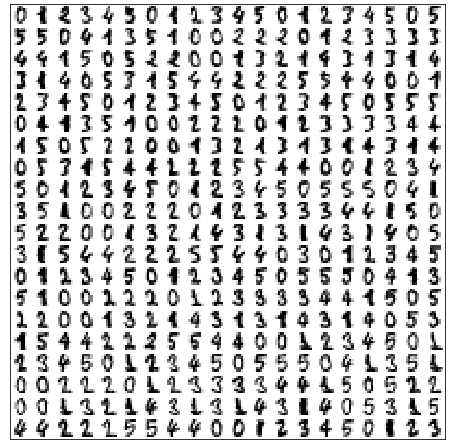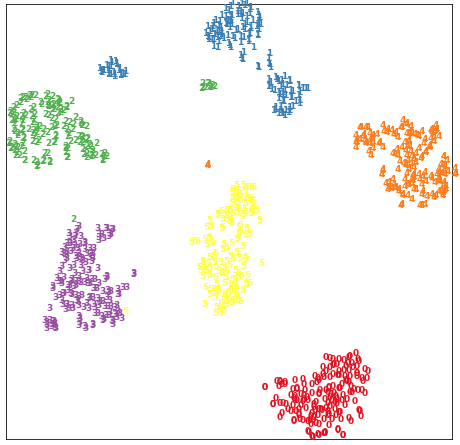# t-SNE实践——sklearn教程

1. 参考sklearn官方文档

# t-SNE

t-SNE是一种集降维与可视化于一体的技术，它是基于SNE可视化的改进，解决了SNE在可视化后样本分布拥挤、边界不明显的特点，是目前最好的降维可视化手段。## TSNE的参数

parameters描述
n_components嵌入空间的维度
perpexity混乱度，表示t-SNE优化过程中考虑邻近点的多少，默认为30，建议取值在5到50之间
early_exaggeration表示嵌入空间簇间距的大小，默认为12，该值越大，可视化后的簇间距越大
learning_rate学习率，表示梯度下降的快慢，默认为200，建议取值在10到1000之间
n_iter迭代次数，默认为1000，自定义设置时应保证大于250
metric表示向量间距离度量的方式，默认是欧氏距离。如果是precomputed，则输入X是计算好的距离矩阵。也可以是自定义的距离度量函数。
init初始化，默认为random。取值为random为随机初始化，取值为pca为利用PCA进行初始化（常用），取值为numpy数组时必须shape=(n_samples, n_components)
verbose是否打印优化信息，取值0或1，默认为0=>不打印信息。打印的信息为：近邻点数量、耗时、 σ σ $\sigma$、KL散度、误差等
random_state随机数种子，整数或RandomState对象
method两种优化方法：barnets_hutexact。第一种耗时O(NlogN)，第二种耗时O(N^2)但是误差小，同时第二种方法不能用于百万级样本
angle当method=barnets_hut时，该参数有用，用于均衡效率与误差，默认值为0.5，该值越大，效率越高&误差越大，否则反之。当该值在0.2-0.8之间时，无变化。

Atrtributes描述
embedding_嵌入后的向量
kl_divergence_KL散度
n_iter_迭代的轮数

## t-distributed Stochastic Neighbor Embedding(t-SNE)

t-SNE可降样本点间的相似度关系转化为概率：在原空间（高维空间）中转化为基于高斯分布的概率；在嵌入空间（二维空间）中转化为基于t分布的概率。这使得t-SNE不仅可以关注局部（SNE只关注相邻点之间的相似度映射而忽略了全局之间的相似度映射，使得可视化后的边界不明显），还关注全局，使可视化效果更好（簇内不会过于集中，簇间边界明显）。

t-SNE的缺点：

• 计算量大，耗时间是PCA的百倍，内存占用大。
• 专用于可视化，即嵌入空间只能是2维或3维。
• 需要尝试不同的初始化点，以防止局部次优解的影响。

## t-SNE的优化

• perplexity 混乱度。混乱度越高，t-SNE将考虑越多的邻近点，更关注全局。因此，对于大数据应该使用较高混乱度，较高混乱度也可以帮助t-SNE拜托噪声的影响。相对而言，该参数对可视化效果影响不大。
• early exaggeration factor 该值表示你期望的簇间距大小，如果太大的话（大于实际簇的间距），将导致目标函数无法收敛。相对而言，该参数对可视化效果影响较小，默认就行。
• learning rate 学习率。关键参数，根据具体问题调节。
• maximum number of iterations 迭代次数。迭代次数不能太低，建议1000以上。
• angle (not used in exact method) 角度。相对而言，该参数对效果影响不大。

PS：一个形象展示t-SNE优化技巧的网站How to Use t-SNE Effectively.

## 代码

### 例一

import numpy as np
import matplotlib.pyplot as plt
from sklearn import manifold, datasets

X, y = digits.data, digits.target
n_samples, n_features = X.shape

'''显示原始数据'''
n = 20  # 每行20个数字，每列20个数字
img = np.zeros((10 * n, 10 * n))
for i in range(n):
ix = 10 * i + 1
for j in range(n):
iy = 10 * j + 1
img[ix:ix + 8, iy:iy + 8] = X[i * n + j].reshape((8, 8))
plt.figure(figsize=(8, 8))
plt.imshow(img, cmap=plt.cm.binary)
plt.xticks([])
plt.yticks([])
plt.show()'''t-SNE'''
tsne = manifold.TSNE(n_components=2, init='pca', random_state=501)
X_tsne = tsne.fit_transform(X)

print("Org data dimension is {}.
Embedded data dimension is {}".format(X.shape[-1], X_tsne.shape[-1]))

'''嵌入空间可视化'''
x_min, x_max = X_tsne.min(0), X_tsne.max(0)
X_norm = (X_tsne - x_min) / (x_max - x_min)  # 归一化
plt.figure(figsize=(8, 8))
for i in range(X_norm.shape):
plt.text(X_norm[i, 0], X_norm[i, 1], str(y[i]), color=plt.cm.Set1(y[i]),
fontdict={'weight': 'bold', 'size': 9})
plt.xticks([])
plt.yticks([])
plt.show()06-161万+09-309万+
12-191698
07-076741
08-081万+
01-306676
04-298413
05-131万+
05-241286
04-0596
12-05110
12-141万+
04-073666
01-19
08-281万+
01-31139
08-316941
01-101823